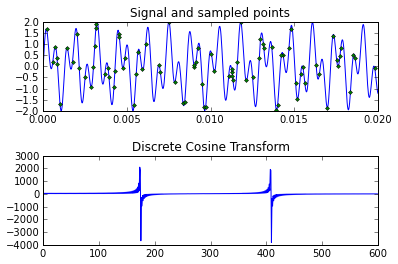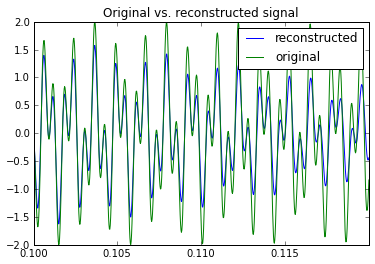# Compressed Sensing Example¶

This workbook follows Cleve Moler's write-up of compressed sensing from http://www.mathworks.com/company/newsletters/articles/clevescorner-compressed-sensing.html

### Background¶

To apply CS, we need to know that our signal can be represented with few non-zero coefficients in some base:

$$\mathbf{f} = \psi \mathbf{x}$$

where $\mathbf{f}$ is the signal, $\psi$ the matrix of basis functions, and $\mathbf{c}$ the coefficients (most of them zero). We start with only a few samples, randomly taken from our signal, i.e.

$$\mathbf{b} = \phi \mathbf{f}$$

where $\phi$ is a decimation matrix (selected rows from the identity matrix). From this, we can write

$$\mathbf{b} = \phi \psi \mathbf{x}.$$

To find $\mathbf{c}$ then requires solving a highly under-determined linear system, $A\mathbf{x}=\mathbf{b}$ with $A=\phi \psi$; but (and this is the secret to CS) we know that most of the saught coefficients should be zero. It therefore seems natural to penalise the solution by the number of non-zeros, but this problem is hard to solve. Instead, Donoho, Candés & Tao showed that penalising with the $\ell_1$-norm is very likely to yield the same result.

We therefore proceed to solve

$$\| A \mathbf{x} - \mathbf{b} \|_2^2 + \lambda \| \mathbf{x} \|_1.$$

In :
import numpy as np
import scipy.fftpack as fft
import matplotlib.pyplot as plt
from sklearn import linear_model


First, we generate a test signal by sampling a sum of two sine waves (the "A" sounds from a touch tone phone):

In :
F = 40000 # Sampling frequency
d = 1./8 # Total sampling duration

T = 1./F # Sampling interval
t = np.linspace(0, d, d / T)

f = np.sin(1394 * np.pi * t) + np.sin(3266 * np.pi * t)


We now set up our sampling and decimation matrices:

In :
# Discrete cosine transform matrix
N = len(f)
C = fft.idct(np.eye(N, N), norm='ortho')

# Decimation operator; randomly choose 500 points
r = np.random.permutation(np.arange(len(f)))
D = np.eye(N, N)[r[:500]]

print "Using %2.1f%% of points." % (len(D) / float(len(f)) * 100)
print "Nyquist rate: %d Hz" % (3266*2)
print "Effective sampling rate: %d Hz" % (len(D) / float(len(f)) * F)

# Form the combined operator
A = D.dot(C)

Using 10.0% of points.
Nyquist rate: 6532 Hz
Effective sampling rate: 4000 Hz

And visualise the effect of those matrices on the signal:

In :
plt.subplot(211)
plt.plot(t, f)
plt.plot(D.dot(t), D.dot(f), '.')
plt.xlim(0, 0.02)
plt.title('Signal and sampled points')

plt.subplot(212)
plt.plot(fft.dct(f)[:600])
plt.title('Discrete Cosine Transform')Let's use scikits.learn to find the $\ell_1$ penalised solution:

In :
L = linear_model.Lasso(alpha=0.001)
b = D.dot(f)
x = L.fit(A, b).coef_

In :
#x, _, _, _ = np.linalg.lstsq(A, b) # minimise BIC criterium to find T
#T = 0.001
#y = (np.abs(x) - T)
#y[y < 0] = 0
#
#x = np.sign(x) * y

# Above would only work for orthogonal A

In :
f_ = C.dot(x)
plt.plot(t, f_, label='reconstructed')
plt.xlim(0.1, 0.12)

plt.plot(t, f, label='original')
plt.title('Original vs. reconstructed signal')
plt.legend();# Notes to self¶

• Change to LassoLars
• Can determine alpha with cross validation
• file bug report: fit doc should have return params: self

L = linear_model.Lasso()
linear_model.LassoCV #  not good for very few non-zeros
-> use this one L = linear_model.LassoLarsCV()
L = linear_model.OMP + nr of nonzeros (not convex, greedy)


In :
b = D.dot(f)

In :
from scikits.learn import svm
svm.l1_min_c(A, b, loss='l2')

Out:
0.001004016064257028
In :